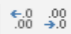# 15.4: Number Group

$$\newcommand{\vecs}{\overset { \rightharpoonup} {\mathbf{#1}} }$$ $$\newcommand{\vecd}{\overset{-\!-\!\rightharpoonup}{\vphantom{a}\smash {#1}}}$$$$\newcommand{\id}{\mathrm{id}}$$ $$\newcommand{\Span}{\mathrm{span}}$$ $$\newcommand{\kernel}{\mathrm{null}\,}$$ $$\newcommand{\range}{\mathrm{range}\,}$$ $$\newcommand{\RealPart}{\mathrm{Re}}$$ $$\newcommand{\ImaginaryPart}{\mathrm{Im}}$$ $$\newcommand{\Argument}{\mathrm{Arg}}$$ $$\newcommand{\norm}{\| #1 \|}$$ $$\newcommand{\inner}{\langle #1, #2 \rangle}$$ $$\newcommand{\Span}{\mathrm{span}}$$ $$\newcommand{\id}{\mathrm{id}}$$ $$\newcommand{\Span}{\mathrm{span}}$$ $$\newcommand{\kernel}{\mathrm{null}\,}$$ $$\newcommand{\range}{\mathrm{range}\,}$$ $$\newcommand{\RealPart}{\mathrm{Re}}$$ $$\newcommand{\ImaginaryPart}{\mathrm{Im}}$$ $$\newcommand{\Argument}{\mathrm{Arg}}$$ $$\newcommand{\norm}{\| #1 \|}$$ $$\newcommand{\inner}{\langle #1, #2 \rangle}$$ $$\newcommand{\Span}{\mathrm{span}}$$$$\newcommand{\AA}{\unicode[.8,0]{x212B}}$$In addition to determining the way that information is displayed in a cell, the user can also determine the format a number is displayed inside a cell. The default display is “General” but selecting the drop-down box allows for many more options for the user to select depending on the cell conditions.Cell Format Function
General Any text or number will display in the cell as written.
Number This allows the user to only display numbers in this field and specifies the number of decimal places.
Currency Similar to Number format, this displays numbers in the cell and the number of decimal places and the chosen currency symbol (depending on the currency) for the user.
Accounting Similar to Currency, Accounting lines up the decimal points in a column so the amounts are displayed with the same alignment.
Short Date Displays a set of numbers as the date, beginning from the year 1900. For example, the number “1” is 1/1/1900
Long Date Displays a set of numbers as the date, beginning from the year 1900. For example, the number “1” is Sunday, January 1, 1900
Time Displays a set of numbers as the time, beginning from the year 1900. For example, the number “1.1” is 2:24:00 AM.
Percentage Displays decimals as a percentage. For example, .2 = 20%
Fraction Displays decimals as a fraction. For example, .2 = 1/5.
Scientific Displays a number as a scientific calculation, for example, .2 = 2.00E^-1
Text Displays the cell information exactly as entered even if a number is displayed.

In addition, students can select more formatting options by selecting the diagonal arrowfor more customization. Below the formatting, the box is some quick shortcut iconsthat allow the user to quickly format a cell with the Accounting (\$), Percentage %, or to add a comma “,” to numbers that have the thousandths place. Finally, to the right of the shortcut icons are decrease indent and increase indent icons. These options allow the user to specify how much numerical detail to place in a cell. As an example, our cell currently contains the numbers 2.1345 as a repeating number which means the decimal will continue to infinity. The cell information goes to the fourth decimal because it was formatted this way by the user (the user can decide how many decimal places to display).

By decreasing the indent to the leftthe number rounds the last decimal up or down depending, and only displays the next decimal place. In the case of this example, 3 decimal places. Therefore, the new number is 2.135 because excel rounded the fourth decimal up. By decreasing the indent to the right,more decimal places will be displayed, and the number becomes 2.13452.

This page titled 15.4: Number Group is shared under a CC BY license and was authored, remixed, and/or curated by Nick Heisserer (Minnesota State Opendora) .